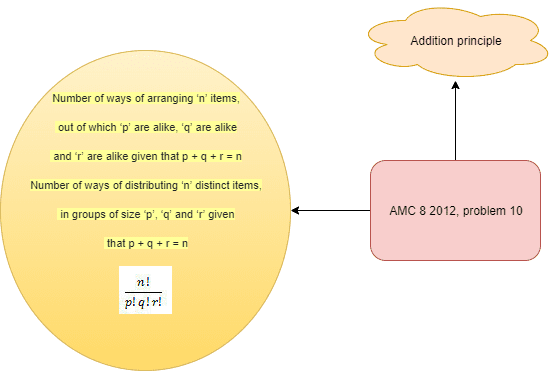Categories

# Permutation and basic counting principle AMC 8 2012, problem 10

Try this beautiful problem from AMC 8. It involves permutation and basic counting principles. We provide sequential hints so that you can try the problem.

# What are we learning ?

Competency in Focus: Basic counting principle and permutation   This problem from American Mathematics contest (AMC 8, 2012)

# First look at the knowledge graph.# Next understand the problem

How many 4-digit numbers greater than 1000 are there that use the four digits of 2012?
##### Source of the problem
American Mathematical Contest 2012, AMC 8 Problem 10
##### Key Competency
Permutation and basic counting principle
4/10
##### Suggested Book
Challenges and Thrills in Pre College Mathematics Excursion Of Mathematics

Do you really need a hint? Try it first!
For this problem, all we need to do is find the amount of valid 4-digit numbers that can be made from the digits of$2012$, since all of the valid 4-digit number will always be greater than$1000$.
The best way to solve this problem is by using casework. Now think what are the cases?
It has two cases , as there can be only two leading digits, namely$1$ or$2$.
We know  that number of ways of arranging ‘n’ items, out of which ‘p’ are alike, ‘q’ are alike and ‘r’ are alike given that p + q + r = n Number of ways of distributing ‘n’ distinct items, in groups of size ‘p’, ‘q’ and ‘r’ given that p + q + r = n. . Now try to calculate the the two cases .
CASE 1: As 2012 consits of two 2’s , one 1, 0 so if we set 1 as the leading digit then we have two twos and one 0 such numbers then we have$\frac{3!}{2!1!} \implies 3$ such numbers.
When the leading digit is$2$ then we have one 2, one 1 and one 0 then we can arrange them in$3! \implies 6$ ways and as such we have 6 such numbers.
By addition principle we find that there are  9 such numbers.

# Similar Problems

## AMC - AIME Program

AMC - AIME - USAMO Boot Camp for brilliant students. Use our exclusive one-on-one plus group class system to prepare for Math OlympiadThis site uses Akismet to reduce spam. Learn how your comment data is processed.